WEBINAR
External Rotor PMSM Technology for Energy Generation and Durability
Thursday, March 30, 2023
Time
SESSION 1
SESSION 2
CEST (GMT +2)
03:00 PM
08:00 PM
EDT (GMT -4)
09:00 AM
02:00 PM
HOME / Applications / Magnetic gear: 2D FEM simulation with EMWorks2D inside SOLIDWORKS

# Magnetic gear: 2D FEM simulation with EMWorks2D inside SOLIDWORKS

Used Tools:## Magnetic Gear

Gears and gearboxes are used to vary the speed and torque transmission in several applications. They are manufactured to match rotational speed/torque of an input source with a required rotational speed/torque of an output load. Traditionally, the mechanical gears have been known to be widely used. However, these systems suffer from some inherent problems such as contact friction, heat, noise and vibration, etc. Reliability and efficiency are reduced significantly because of these problems.

To overcome these issues, new kind of gears are being developed. Magnetic gears which achieve non-contact power transmission, are becoming popular. They offer a frictionless transmission which can save lubrication and maintenance costs and minimize heat issues. Magnetic gears inherently protect against overloads by harmlessly slipping if an overload torque is applied and automatically and safely re-engaging when the fault torque is removed. In addition to less acoustic noise and vibration, the above-mentioned advantages help to obtain a highly efficient and safe gear systems. Hence, these contactless power transmission devices can be found in the following applications : overload protection applications requiring high levels of safety (cordless power tools, factory conveying equipment, etc.), clean room applications requiring no metal powders  (wafer-transfer robots, wafer steppers and semiconductor step-and-scan systems), powered mobility applications requiring levels of efficiency (transmission systems for electric bikes, scooters, etc.). Several structures and topologies of magnetic gear are studied and developed depending on their roles and geometries (coaxial, parallel axial, passive, active, etc.). Figure 1 below shows some corresponding gear topologies between mechanical and magnetic gears .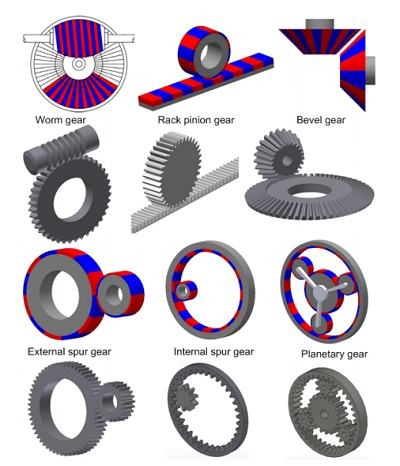Figure 1 - Magnetic gear topologies

However, magnetic gears have some disadvantages such as large size, magnetic field interference, expensive permanent magnets, low torque density, constant power ratio, etc. Thus, both industry and researchers are working to improve and enhance magnetic gears and overcome these disadvantages. The magnetic flux and the transferred torque are two important factors in designing and optimizing magnetic gears.

Finite element method (FEM) has demonstrated high reliability in the design and analysis of engineering systems . It can be used to simulate any complicated geometry and for both linear and nonlinear problems. Moreover, Finite element analysis (FEA) can be used to solve both 3D and 2D approximated geometry. Additional advantages are gained when using 2D FEA such as saving time, running more iterations, etc. EMWorks2D, which is a 2D FEM plug-in inside SOLIDWORKs, provides an accurate solution to magnetic and electric problems in cases where the real life problem can be approximated as a 2D problem.

In this article, EMWorks2D is used to compute magnetic results (magnetic flux density, field intensity, torque, etc.) of two coaxial magnetic gear models. Torque results will be compared to the reference results.

## 2D simulation of coaxial magnetic gear 

A coaxial magnetic gear model is presented in . It consists of a Pi= 2 pole-pairs inner rotor, a Po=3 pole-pairs outer rotor and Q=5 ferromagnetic pole-pieces. The inner rotor rotates with higher speed than the outer rotor. Thus, the inner rotor is a high-speed rotor (HS) and the outer rotor is a low-speed rotor (LS). The LS rotor develops higher torque than the HS rotor. The magnetic flux in the air gap region and the developed torque is evaluated using EMWorks2D. End effects are neglected in this analysis. The permeability of iron cores is infinite while the remanence and the relative recoil permeability of the magnets are respectively 1.2T and. The magnets have an alternating radial magnetization.

The model is built inside SOLIDWORKS. Table 1 groups the main properties of the magnetic gear model. Figure 2 shows the 2D model created with SOLIDWORKS.

Table 2 - Main properties of the MG
 Items Value Radius of the inner rotor yoke 40.00 mm Outer radius of the inner rotor PMs 50.00 mm Inner radius of the slots 52.00 mm Outer radius of the slots 62.00 degree Slot opening 36.00 deg Inner radius of the outer rotor PMs 64.00 mm Inner radius of the outer rotor yoke 74.00 mm Axial length 100.00 Pole-pairs inner rotor 2 Pole-pairs outer rotor 3 Ferromagnetic pole-pieces 5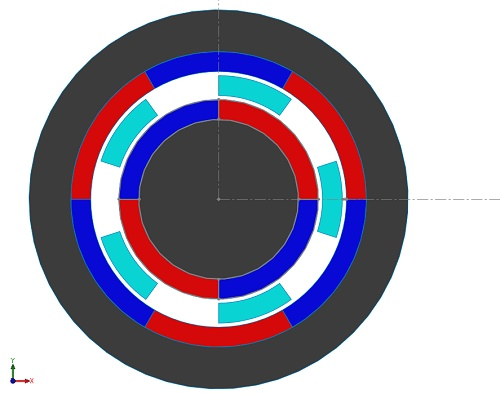Figure 2 - 2D model of the simulated magnetic gear system

EMWorks2D is equipped with an automatic and flexible mesher that generates triangular mesh elements. A mesh refinement is applied on the air gap region to get accurate results. Figure 3 shows the mesh plot created by EMWorks2D.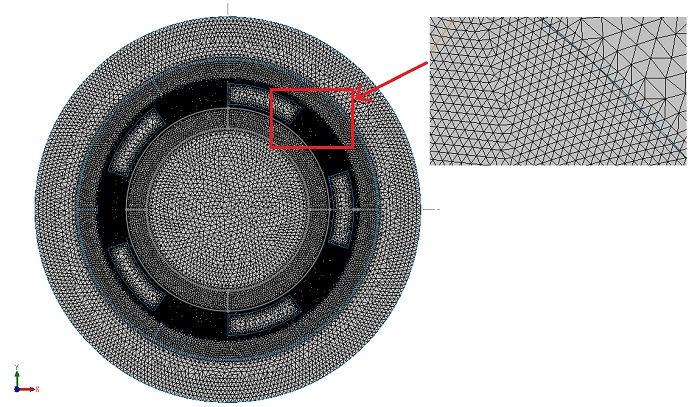Figure 3 - Plot of the mesh created in EMWORKS 2D

Initially, the LS rotor and the ferromagnetic pieces are fixed while the HS rotor is spinning with an angleranging from 0° to 90° . A parametric sweep analysis is performed with EMWorks2D to compute the magnetic flux and the torque results versus the inner rotor angle. Figures 4a) and 4b) show the magnetic flux density distribution inside the model respectively atand. The plots contain high flux spots in the ferromagnetic bodies. Figure 5 illustrates the magnetic flux density in the air gap region at the angle. It shows a non-homogeneous distribution due to the positions of the poles of the inner and outer rotors. The magnetic vector potential lines are plotted in Figure 6. The ferromagnetic pieces help to conduct the magnetic flux in the intermediate air region between the HS and LS rotors poles. Practically, all the magnetic flux density is circulating inside the magnetic gear device as can be seen in Figure 6.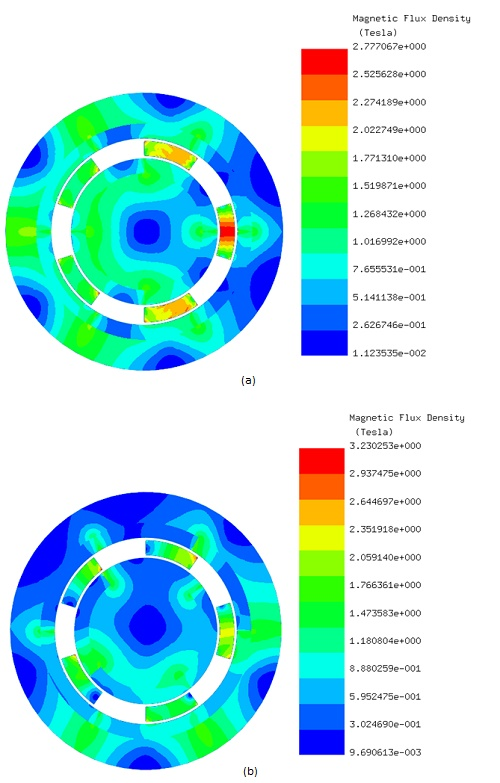Figure 4 - Magnetic flux results, a)b)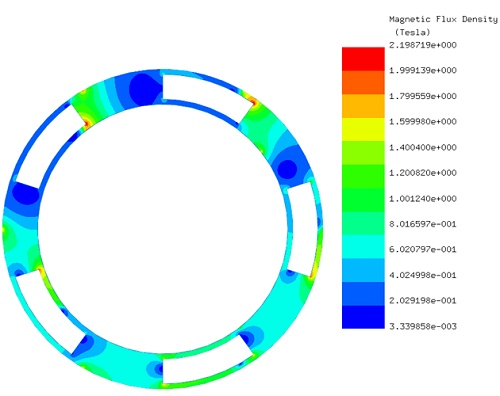Figure 5 - Magnetic flux density in the air gap region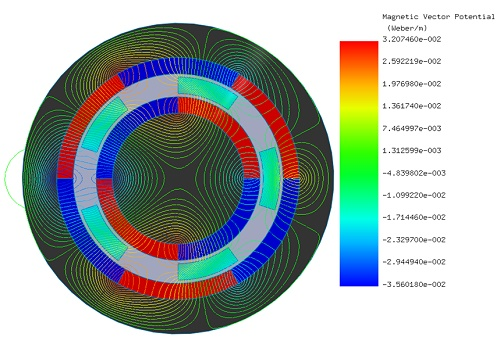Figure 6 - Magnetic vector potential lines ()

Figure 7 shows the magnetic torque generated on the HS rotor while the LS rotor and the ferromagnetic pieces are kept stationary. The torque is nearly null at, then it starts increasing with the angle until reaching a first peak of 70 N.m at. The torque curve reaches a second peak of 74 N.m at, the it drops down until becoming again null at.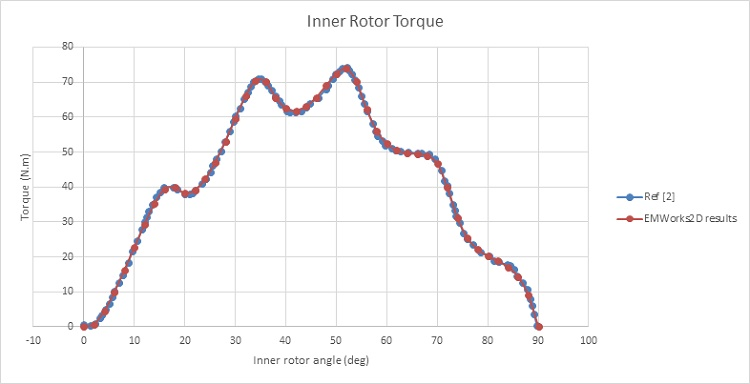Figure 7 - HS rotor torque (LS rotor and ferromagnetic pieces are fixed)

Now the generated torques are evaluated when the inner and outer rotors are both rotating in opposite direction (ferromagnetic pieces are fixed).

Outer rotor angleis described using the following equation:. The starting position of  the HS rotor iswhich corresponds to a torque value around 62 N.m while it is about 98 N.m in the LS rotor. Figures 8a) and 8b) represent the exerted torques respectively in the inner and the outer rotors. The mean torque generated in HS rotor is around 67 N.m while it is nearly 101 N.m in the LS rotor which leads to a gear ratio around 3:2. Hence If the inner rotor is rotated, then the torque is amplified 1.5 times by this magnetic gear system.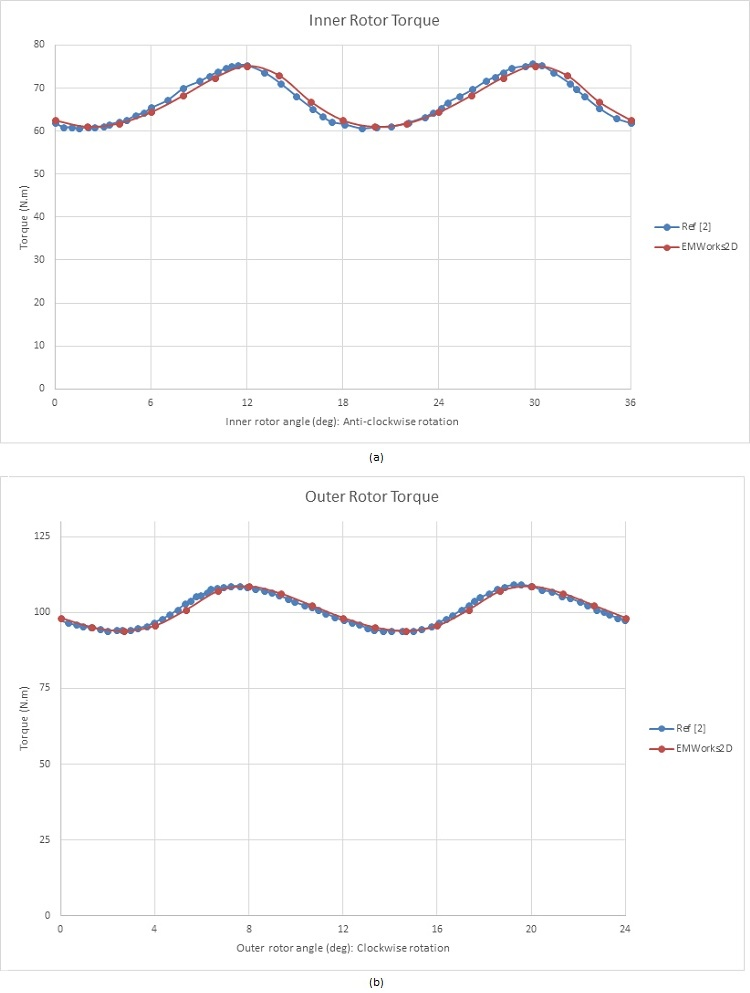Figure 8 - Torque results, a) inner rotor, b) outer rotor

The above torque curves are characterized by high ripples. To overcome this issue, a new model with higher pole-pairs is developed and studied. The new magnetic gear structure  is shown in Figure 9. It contains Pi=3 inner rotor pole-pairs while the outer rotor has Po=13 pole pairs. This leads to a gear ratio equal to 4.33. The ferromagnetic pieces number is Q=16.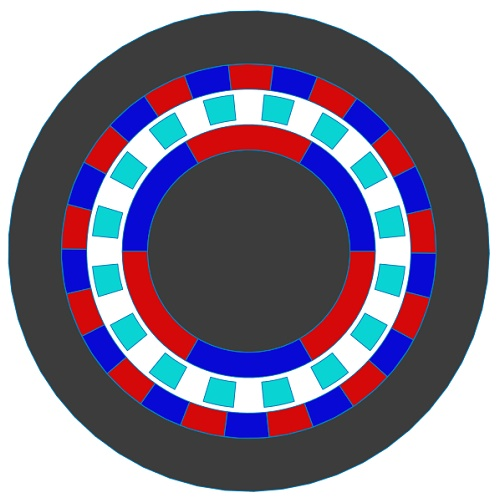Figure 9 - 2D model of the new magnetic gear system

The flux lines are plotted in Figure 10. The intermediate ferromagnetic pieces conduct the flux in the air gap region. As in the previous section, the first torque curve is computed when the HS rotor rotates while LS rotor and ferromagnetic pieces are kept fixed (varies from 0° to 60°). The calculated torque of the HS rotor is shown in Figure 11. It has a maximum value around 41 N.m at. Figures 12a) and 12b) give the torque curves generated respectively in the inner rotor and the outer rotor while they are spinning in opposite direction. The HS rotor develops a Torque of 41 N.m while the generated torque in LS rotor is about 175 N.m. Thus, both torques verify the calculated gear ratio and are characterized by lower ripples. As can be seen from Figure 12b), the ripples are practically eliminated.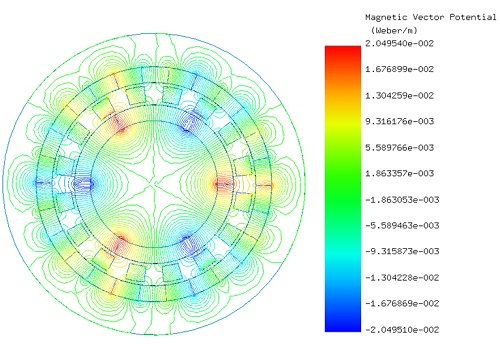Figure 10 - Flux lines plot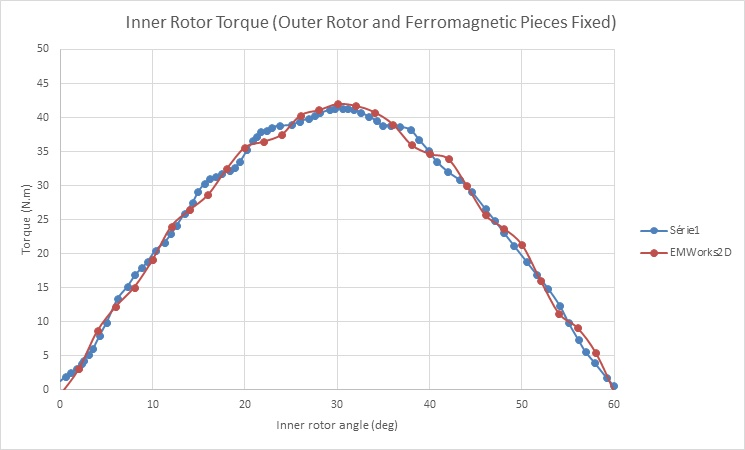Figure 11 - Inner rotor torque while keeping outer rotor and ferromagnetic pieces fixed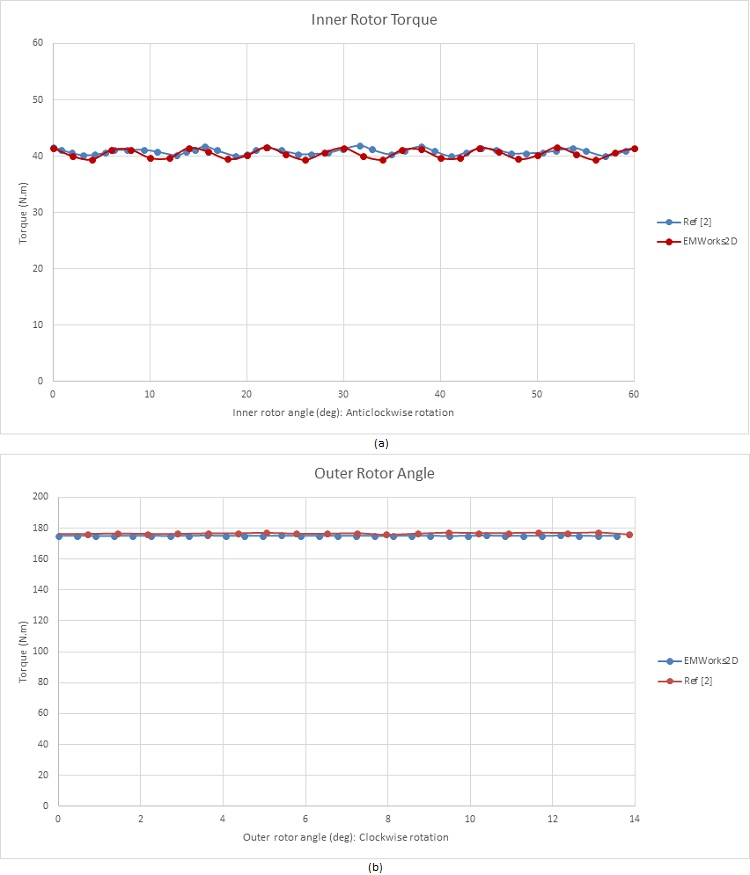Figure 12 - Torque results, a) inner rotor, b) outer rotor

## Conclusion

Magnetic gear systems have shown high capabilities in power transmission due to several advantages it presents. Thus, they replaced traditional mechanical gears in many applications and fields. However, they are still suffering from some issues and limitations. Computer simulations are used to study and develop new magnetic gears offering higher efficiency and stability. Numerical simulation helps to accelerate the development process of these magnetic gears by saving time and money by reducing prototyping and give accurate data in real life conditions. EMWorks2D was used in this article to predict the magnetic results (magnetic flux and torque).

## References

:P.M. Tlali, R-J. Wang, S. Gerber. Magnetic Gear Technologies: A Review. IEEE: 2014 International Conference on Electrical Machines (ICEM)
:Thierry Lubin, Smail Mezani, Abderrezak Rezzoug. Analytical Computation of the Magnetic Field Distribution in a Magnetic Gear.
Groupe de Recherche en Electrotechnique et Electronique de Nancy, University Henri Poincaré, Nancy, FRANCE